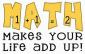# detailed solution please? total energy of rotating rod

2 posts / 0 new
Juny Geraldizo ...detailed solution please? total energy of rotating rod

the rod is 10ft long weighing 20lbs. if it revolves through one end at 2rps, find the total energy.

Jhun VertUse the formula for kinetic energy of rotating rod:
KE = energy due to rotation + energy due to translation of center of mass
$KE = \frac{1}{2}I\omega^2 + \frac{1}{2}mv^2$

• Mathematics inside the configured delimiters is rendered by MathJax. The default math delimiters are $$...$$ and $...$ for displayed mathematics, and $...$ and $...$ for in-line mathematics.